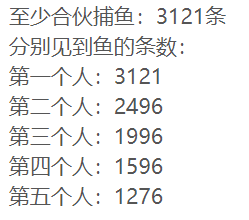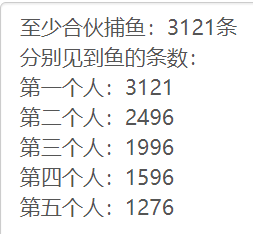# C语言 | 两种方法解决五人分鱼

A、B、C、D、E 五人在某天夜里合伙去捕鱼，到第二天凌晨时都疲惫不堪，于是各自找地方睡觉。

B 第二个醒来，也将鱼分为五份，把多余的一条鱼扔掉拿走自己的一份。 。

C、D、E依次醒来，也按同样的方法拿鱼。

### 方法一

#include <stdio.h>
int main(){
int n,x,j,k,l,m;
for(n=5;;n++){
j=4*(n-1)/5;
k=4*(j-1)/5;
l=4*(k-1)/5;
m=4*(l-1)/5;
if(n%5==1&&j%5==1&&k%5==1&&l%5==1&&m%5==1){
printf("至少合伙捕鱼：%d条\n",n);
printf("分别见到鱼的条数：\n第一个人：%d \n第二个人：%d \n第三个人：%d \n第四个人：%d\n第五个人：%d \n",n,j,k,l,m);
break;
}
}
return 0;
}### 方法二

#include <stdio.h>
int main(){
int n,x,j,k,l,m;
for(n=5;;n++){
j=5*n/4+1;
k=5*j/4+1;
l=5*k/4+1;
m=5*l/4+1;
if(n%5==1&&j%5==1&&k%5==1&&l%5==1&&m%5==1){
printf("至少合伙捕鱼：%d条\n",m);
printf("分别见到鱼的条数：\n第一个人：%d \n第二个人：%d \n第三个人：%d \n第四个人：%d\n第五个人：%d \n",m, l, k, j, n);
break;
}
}
return 0;
}01-11423406-232万+
07-133132
08-182万+
04-234万+
09-17401
04-11536
08-22178
10-166万+
09-112万+
06-211万+
05-24322
03-07999
11-0525
08-04968
04-01645
©️2020 CSDN 皮肤主题: 大白 设计师:CSDN官方博客